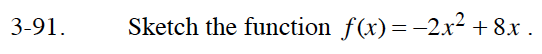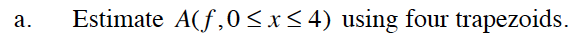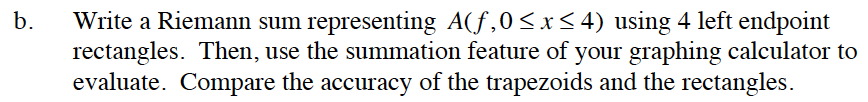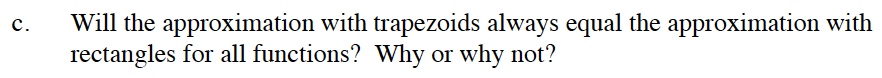### Home > CALC > Chapter Ch3 > Lesson 3.3.1 > Problem3-91

3-91.$\text{Area of a trapezoid }=\frac{1}{2}h(b_1+b_2).$

On the given domain, what will be the height of each trapezoid?

The bases are determined by the function.1[f(0) + f(1) + f(2) + f(3)]